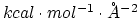Next: Runtime Analysis Up: Hybrid MD-Go Simulation Previous: Configuration file modifications   Contents   Index

## GoParameter format

When running a Go simulation, the atoms are partitioned into chains according to the occupancy value given in the GoCoordinates file. For every possible pairwise combination between chains, a Go potential is defined by the following equations:

Letbe the pairwise distance between atoms i and j in the reference structure. Ifis less than the Go cutoff distance, the pairwise potential between atoms i and j is given by:whereis given as. Ifis greater than the Go cutoff distance, the pairwise potential between atoms i and j is given by:For each pairwise chain combination, the following parameters are needed to define the Go potential:

• chaintypes (2 floats): (first_chain second_chain) Defines the pairwise chain interaction
• epsilon (1 float): () Determines theconstant of the Go potential in units of• exp_a (1 integer): (a) Determines the a' constant for the Go potential
• exp_b (1 integer): (b) Determines the b' constant for the Go potential
• expRep (1 integer): (expRep) Determines the `expRep' constant for the Go potential
• sigmaRep (1 float): () Determines theconstant for the Go potential in units of• epsilonRep (1 float): () Determines theconstant for the Go potential in units of• cutoff (1 float): (cutoff) Defines the Go cutoff distance for this particular pairwise chain in units of• [Optional] restriction (1 integer): Determines if interactions between theandadjacent residue should be excluded. Multiple restriction between adjacent residues can be defined within a chaintype. Each additional new restriction is given on its own line.

Each pairwise chaintype should be written in its own block of text with each entry given its own line. It is recommended that individual pairwise potential be separated by a blank line.Next: Runtime Analysis Up: Hybrid MD-Go Simulation Previous: Configuration file modifications   Contents   Index
http://www.ks.uiuc.edu/Research/namd/